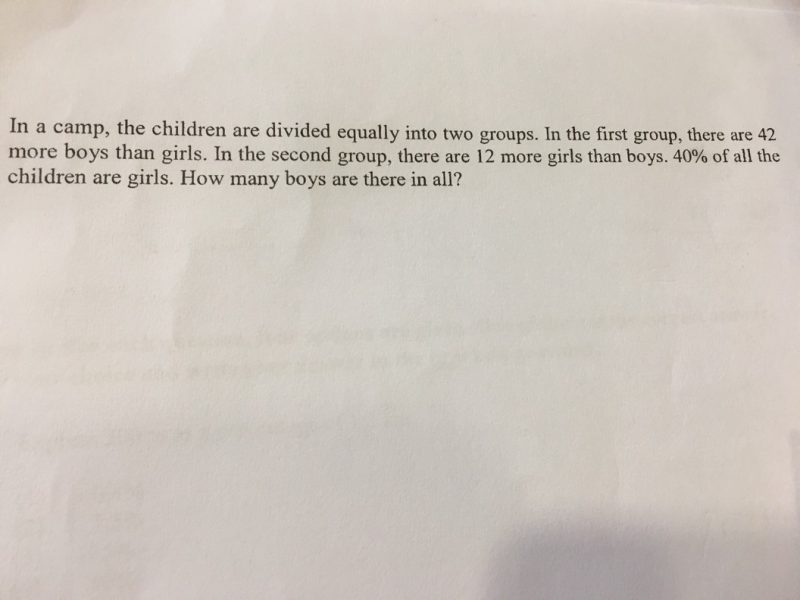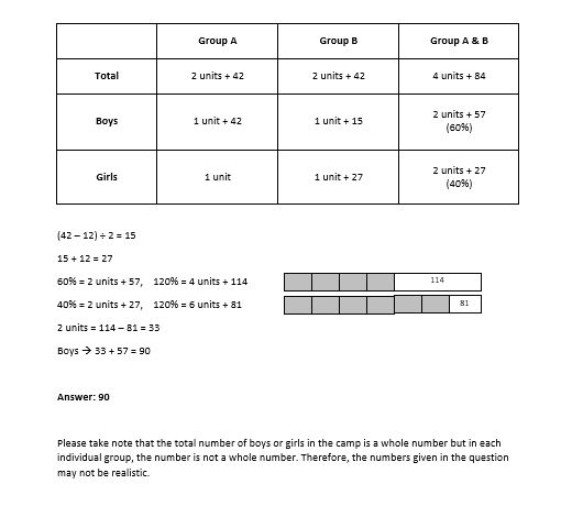# Question1st group —
girls : 1 unit
boys : 1 unit + 42
total : 2 units + 42

2 units + 42 – 12 = 2 units + 30
(2 units + 30)/2 = 1 unit + 15 (boys in 2nd group)
1 unit + 15 + 12 = 1 unit + 27 (girls in 2nd group)

2nd group —
girls : 1 unit + 27
boys : 1 unit + 15
total : 2 units + 42

total girls ——-> 1 unit + 1 unit + 27 = 2 units + 27
total boys ——-> 1 unit + 42 + 1 unit + 15 = 2 units + 57

(2 units + 27)/(2 units + 57) = 4/6
12 units + 162 = 8 units + 228
1 unit = 16.5
2 units + 57 = 90

Ans : 90 boys.
Note : The question is flawed as 1 unit = 16.5.

0 Replies 0 Likes0 Replies 0 Likes